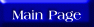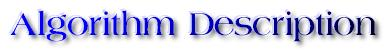The mergesort algorithm closely follows the divide-and-conquer paradigm. Generally, Merge Sort is considered to be one of the best internal sorting methods. If the data is too large to be accommodated all at once in the memory then one has to use external sorting methods. In external sorting methods small blocks of data are loaded into the memory, sorted using an internal sorting method and written out to a tape or disk. Sorted parts are then merged using variations of the merging algorithm discussed below:

Mergesort(A,p,r){
if p < r
then q <- |(p+ r)/2|
Mergesort(A,p,q)
Mergesort(A,q+1,r)
Merge(A,p,q,r)
}

To sort the entire sequence A, Mergesort(A,1,length[A]) is called where length[A]=N.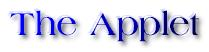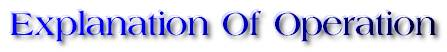Mergesort works by splitting the list into two halves and then sorting each half recursively. Mergesort may be considered to be a tree based algorithm and over here it is executed in a depth first manner. For the above list the algorithm starts by handling the merging of elements as follows (the trend in which the sublists are being considered):

1. First, elements 1 and 2 are handled.
2. Then, elements from 0 through 2 are handled (note the black items indicating the border elements).
3. Then, elements 3 and 4 are handled. After this, elements 5 and 6.
4. Then, elements 3 through 6 are handled.

Subsequently, elements 0 through 6 are handled and so on until elements 0 to 14 are handled.

The Merge algorithm takes two sorted lists and merges them to give a sorted list. It assumes the existence of a temporary merge space whose current contents are shown on the upper canvas of the above applet. Mereg takes the the smaller of the first elements of the two lists and places it into the merge space. When either input list is exhausted, the remainder of the other list is copied to the merge space. The sorted sublist is then copied back from the merge space into the appropriate indices.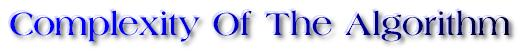Merge Sort algorithm uses the process of merging two sorted lists recursively to accomplish the sorting of an unsorted list. Firstly, two sorted lists of length M and N respectively can be merged in O(M+N) time. If the lists have the same size N then the time will be O(2*N) or O(N).
First, each individual element of the list is considered as a 1-element sorted list. The merge algorithm is then used N/2 times to merge pairs of elements into sorted lists of length 2. This will produce N/2 sorted lists each of length 2. It takes N/2 comparisons to accomplish this. Pairs of 2-element lists are then merged to produce N/4 sorted lists each of length 4. In this case there are N/4 merge operations each taking at most 4 comparisons. This process continues until we have two sorted lists of size N/2 and we merge them in O(N) time. There are log(N) levels of merging. Hence, Merge Sort has a worst-case complexity of O(N*log(N)).